# Solved 2023 Question Paper ICSE Class 10 Chemistry

## SECTION A (40 Marks)

#### Question 1(i)

An element in period 3, whose electron affinity is zero:

1. Neon
2. Sulphur
3. Sodium
4. Argon

Argon

Reason — Argon belongs to period 3. It is an inert element and its octet is complete hence, it's electron affinity is zero.

#### Question 1(ii)

An element with the largest atomic radius among the following is:

1. Carbon
2. Nitrogen
3. Lithium
4. Beryllium

Lithium

Reason — The given elements belong to the second period and Lithium is the first element of second period. Atomic size decreases from left to right in a period hence, Lithium will have the largest atomic radius.

#### Question 1(iii)

The compound that is not an ore of aluminium:

1. Cryolite
2. Corundum
3. Fluorspar
4. Bauxite

Fluorspar

Reason — Fluorspar is calcium fluoride [CaF2] whereas Bauxite [Al2O3.2H2O], Corundum [Al2O3] and Cryolite [Na3AlF6] are ores of aluminium.

#### Question 1(iv)

The vapour density of CH3OH is ............... (At. Wt. C=12, H=1, O=16)

1. 32
2. 18
3. 16
4. 34

16

Reason

Molecular mass of methyl alcohol [CH3OH] = 12 + 3(1) + 16 + 1 = 32

Vapour density = $\dfrac{\text{molecular mass}}{2}$ = $\dfrac{32}{2}$ = 16

#### Question 1(v)

Which of the following reactions takes place at the anode during the electroplating of an article with silver?

1. Ag - 1e- ⟶ Ag1+
2. Ag + 1e- ⟶ Ag1-
3. Ag - 1e- ⟶ Ag
4. None of the above

Ag - 1e- ⟶ Ag1+

Reason — During electroplating of an article with silver, the silver anode loses electrons to give Ag1+ ions in the solution.

#### Question 1(vi)

The metallic hydroxide which forms a deep inky blue solution with excess ammonium hydroxide solution is:

1. Fe(OH)2
2. Cu(OH)2
3. Ca(OH)2
4. Fe(OH)3

Cu(OH)2

Reason — Cu(OH)2 reacts with a solution of excess ammonium hydroxide to form a deep blue solution of tetrammine copper [II] sulphate.

Cu(OH)2 + (NH4)2SO4 + 2NH4OH ⟶ [Cu(NH3)4]SO4 + 4H2O

#### Question 1(vii)

An example of a cyclic organic compound is:

1. Propene
2. Pentene
3. Butene
4. Benzene

Benzene

Reason — In benzene, six carbon atoms form a ring structure and there are three single and three double bonds between the carbon atoms. Hence, benzene is a cyclic compound.

#### Question 1(viii)

In the laboratory preparation, HCl gas is dried by passing through:

1. dilute nitric acid
2. concentrated sulphuric acid
3. dilute sulphuric acid
4. acidified water

concentrated sulphuric acid

Reason — Concentrated Sulphuric acid only removes the moisture content of HCl gas but does not react with it. Hence, it is used as a drying agent for HCl gas.

#### Question 1(ix)

The nitrate which on thermal decomposition leaves behind a residue which is yellow when hot and white when cold:

2. Ammonium nitrate
3. Copper nitrate.
4. Zinc nitrate

Zinc nitrate

Reason

$\begin{matrix} 2\text{Zn(NO}_3)_2 &\longrightarrow & \text{2ZnO} & + & 4\text{NO}_2 &+ & \text{O}_2 \\ \text{[Zinc} & & \text{[Zinc nitrate - white]} & & \text{[Carbon} \\ \text{carbonate]} & & \text{[yellow - hot]} & & \text{dioxide]} \\ \text{[white]} & & \text{[white - cold]} \end{matrix}$

Zinc nitrate decomposes to produces zinc oxide, which is yellow when hot and white when cold

#### Question 1(x)

The salt formed when concentrated sulphuric acid reacts with KNO3 above 200°C:

1. K2SO4
2. K2SO3
3. KHSO4
4. KHSO3

K2SO4

Reason

$\text{KNO}_3 + \text{H}_2\text{SO}_4 [\text{conc.}]\xrightarrow{\gt 200 \degree\text{C}} \text{K}_2\text{SO}_\text{4} + \text{HNO}_3$

#### Question 1(xi)

The property exhibited by concentrated sulphuric acid when it is used to prepare hydrogen chloride gas from potassium chloride:

1. Dehydrating property
2. Drying property
3. Oxidizing property
4. Non-volatile acid property

Non-volatile acid property

Reason — Concentrated sulphuric acid has a high boiling point, hence it is used to prepare hydrogen chloride gas from potassium chloride.

#### Question 1(xii)

The hydrocarbon formed when sodium propanoate and soda lime are heated together:

1. Methane
2. Ethane
3. Ethene
4. Propane

Ethane

Reason

$\underset{\text{sodium propionate}}{\text{C}\text{H}_3\text{-C}\text{H}_2\text{.COONa}} + \underset{\text{sodalime}}{\text{NaOH}} \xrightarrow[\Delta]{\text{CaO}} \underset{\text{ethane}}{\text{C}_2\text{H}_6} + \text{Na}_2\text{CO}_3$

#### Question 1(xiii)

The acid which does not form acid salt by a basic radical:

1. H2CO3
2. H3PO4
3. H2SO4
4. CH3COOH

CH3COOH

Reason — Acetic acid is a weak acid which does not dissociate completely in water, so it does not form acid salt but forms a normal salt.

#### Question 1(xiv)

The general formula of hydrocarbons with single covalent bonds is:

1. CnH2n+2
2. CnH2n
3. CnH2n-2
4. CnH2n-6

CnH2n+2

Reason — The general formula of hydrocarbons with single covalent bonds is CnH2n+2

#### Question 1(xv)

The indicator which changes to pink colour in an alkaline solution is:

1. Blue Litmus
2. Methyl Orange
3. Red Litmus
4. Phenolphthalein

Phenolphthalein

Reason — Colourless phenolphthalein changes to pink colour in an alkaline solution.

#### Question 2(i)

Match the Column A with Column B:

Column AColumn B
(a) Sodium Chloride1. has two shared pair of electrons
(b) Methane2. has high melting and boiling points
(c) Hydrogen chloride gas3. a greenhouse gas
(d) Oxidation reaction4. has low melting and boiling points
(e) Water5. Zn - 2e- ⟶ Zn2+
6. S + 2e- ⟶ S2-

Column AColumn B
(a) Sodium Chloride2. has high melting and boiling points
(b) Methane3. a greenhouse gas
(c) Hydrogen chloride gas4. has low melting and boiling points
(d) Oxidation reaction5. Zn - 2e- ⟶ Zn2+
(e) Water1. has two shared pair of electrons

#### Question 2(ii)

The following sketch illustrates the process of conversion of Alumina to Aluminium:

Study the diagram and answer the following: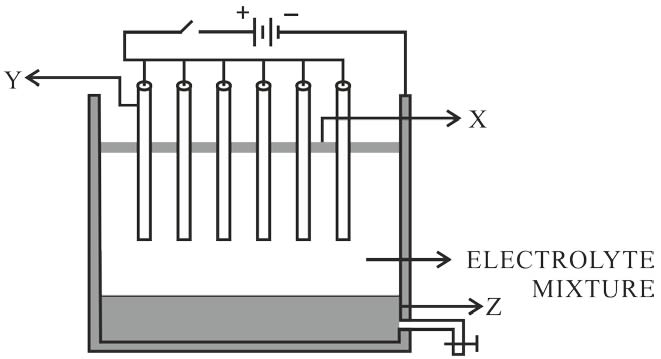(a) Name the constituent of the electrolyte mixture which has a divalent metal in it.

(b) Name the powdered substance 'X' sprinkled on the surface of the electrolyte mixture.

(c) What is the name of the process?

(d) Write the reactions taking place at the electrodes 'Y' (anode) and 'Z' (cathode) respectively.

(a) Fluorspar [CaF2]

(b) Powdered coke

(c) Hall-Heroult's process

(d) Reactions taking place at the electrodes 'Y' (anode) and 'Z' (cathode):

At anode:
6O2- - 12e- ⟶ 6[O]
3O + 3O ⟶ 3O2

At cathode:
4Al3+ + 12e- ⟶ 4Al

#### Question 2(iii)

Fill in the blanks with the choices given in the brackets:

(a) Metals are good ............... [oxidizing agents /reducing agents]

(b) Non-polar covalent compounds are ............... [good / bad] conductors of heat and electricity.

(c) Higher the pH value of a solution, the more is ............... [acidic / alkaline] it is.

(d) ............... [Silver chloride / Lead chloride] is a white precipitate that is soluble in excess of Ammonium hydroxide solution.

(e) Conversion of ethene to ethane is an example of ............... . [hydration / hydrogenation]

(a) Metals are good reducing agents

(b) Non-polar covalent compounds are bad conductors of heat and electricity.

(c) Higher the pH value of a solution, the more alkaline it is.

(d) Silver chloride is a white precipitate that is soluble in excess of Ammonium hydroxide solution.

(e) Conversion of ethene to ethane is an example of hydrogenation.

#### Question 2(iv)

State the terms/process for the following:

(a) The energy released when an atom in the gaseous state accepts an electron to form an anion.

(b) Tendency of an element to form chains of identical atoms.

(c) The name of the process by which Ammonia is manufactured on a large scale.

(d) A type of salt formed by partial replacement of hydroxyl radicals with an acid radical.

(e) The ratio of the mass of a certain volume of gas to the same volume of hydrogen measured under the same conditions of temperature and pressure.

(a) Electron affinity

(b) Catenation

(c) Haber’s process

(d) Basic salt

(e) Vapour Density

#### Question 2(v)

(a) Give the structural formula of the following organic compounds:

1. 2-chlorobutane
2. Methanal
3. But-2-yne

(b) Give the IUPAC name of the following organic compounds: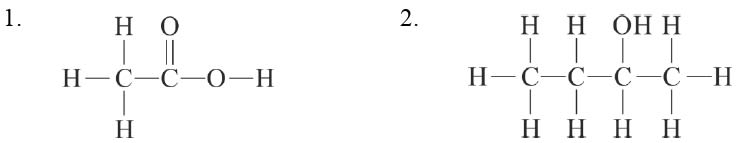(a) Structural formula

1. 2-chlorobutane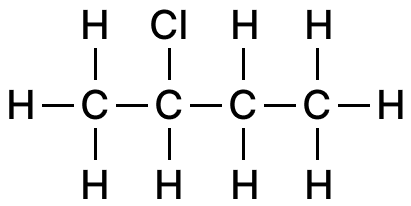2. Methanal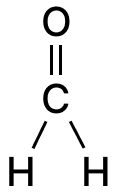3. But-2-yne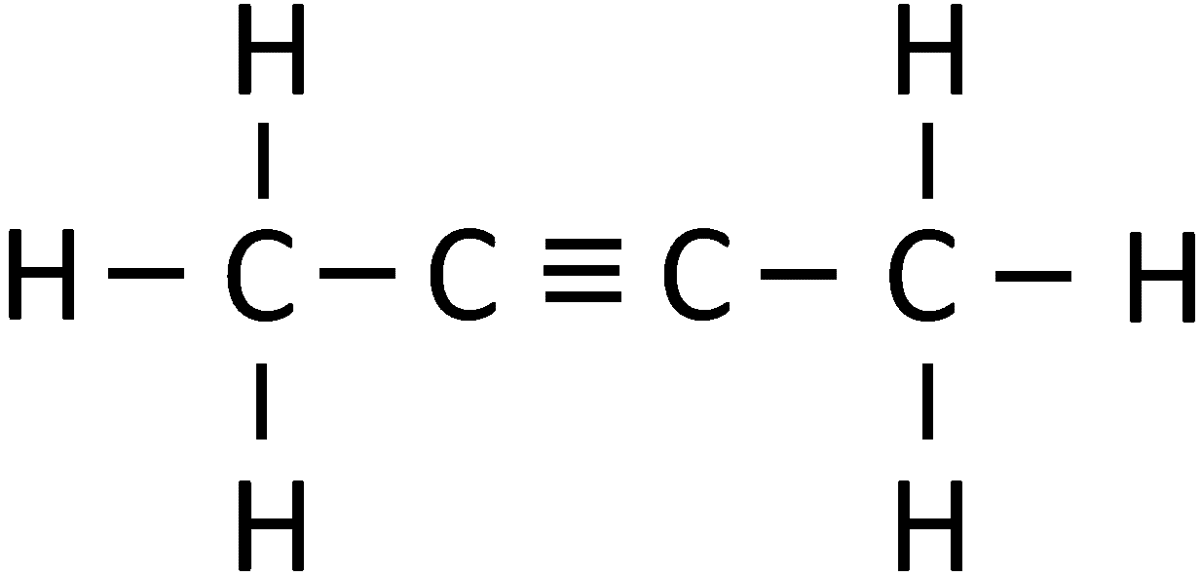(b) IUPAC names are:

1. Ethanoic acid
2. Butan-2-ol

## SECTION B (40 MARKS)

#### Question 3(i)

Identify the cation in each of the following cases:

(a) Ammonium hydroxide solution when added to Solution B gives a white precipitate which does not dissolve in excess of ammonium hydroxide solution.

(b) Sodium hydroxide solution when added to Solution C gives a white precipitate which is insoluble in excess of sodium hydroxide solution.

(a) Pb2+

Reason — When ammonium hydroxide solution is added to lead nitrate, it forms a chalky white precipitate of lead hydroxide [Pb(OH)2] which is insoluble in excess of ammonium hydroxide.

Pb(NO3)2 + 2NH4OH ⟶ 2NH4NO3 + Pb(OH)2

(b) Ca2+

Reason — When sodium hydroxide solution is added to calcium nitrate, a white precipitate of calcium hydroxide is obtained which is insoluble in excess of sodium hydroxide solution.

Ca(NO3)2 + 2NaOH ⟶ Ca(OH)2 ↓ + 2NaNO3

#### Question 3(ii)

Fill in the blanks by choosing the correct answer from the brackets:

(a) During electrolysis, the compound ............... in its molten state liberates reddish brown fumes at the anode. [NaCl/PbBr2]

(b) The ion which could be discharged most readily during electrolysis is ............... [Fe2+/Cu2+]

(a) During electrolysis, the compound PbBr2 in its molten state liberates reddish brown fumes at the anode.

(b) The ion which could be discharged most readily during electrolysis is Cu2+

#### Question 3(iii)

Arrange the following as per the instruction given in the brackets:

(a) Al, K, Mg, Ca (decreasing order of its reactivity)

(b) N, Be, O, C (increasing order of non-metallic character)

(c) P, Si, F, Be (decreasing order of valence electrons)

(a) K > Ca > Mg > Al
Reason — According to the electrochemical series metals at the top of the series are most reactive and the reactivity decreases down the group.

(b) Be < C < N < O
Reason — Across a period, left to right in a periodic table, the non-metallic character increases.

(c) F > P > Si > Be
Reason — The valence electrons decreases from right to left in the periodic table.

#### Question 3(iv)

Complete and balance the following equations:

(a) NH4Cl + Ca(OH)2

(b) CuSO4 + NH4OH ⟶

(c) Cu + Conc. HNO3

(a) 2NH4Cl + Ca(OH)2 ⟶ CaCl2 + 2NH3 + 2H2O

(b) CuSO4 + 2NH4OH ⟶ (NH4)2SO4 + Cu(OH)2

(c) Cu + 4HNO3 (conc.) ⟶ Cu(NO3 )2 + 2NO2 + 2H2O

#### Question 4(i)

State a relevant reason for the following:

(a) Hydrogen chloride gas cannot be dried over quick lime.

(b) Ammonia gas is not collected over water.

(a) Quicklime [CaO] is alkaline in nature and it reacts with HCl forming the respective chloride. Hence, it can't be used as a drying agent for Hydrogen chloride gas.

CaO + 2HCl ⟶ CaCl2 + H2O

(b) As ammonia gas is highly soluble in water, therefore, it is not collected over water.

#### Question 4(ii)

Identify the alloy in each case from the given composition:

(a) aluminium, magnesium, manganese, copper

(b) iron, nickel, chromium, carbon

(a) Duralumin

(b) Stainless steel

#### Question 4(iii)

Solve the following numerical problem.

Ethane burns in oxygen according to the chemical equation:

2C2H6 + 7O2 ⟶ 4CO2 + 6H2O

If 80 ml of ethane is burnt in 300 ml of oxygen, find the composition of the resultant gaseous mixture when measured at room temperature.

[By Lussac's law]

$\begin{matrix} 2\text{C}_2\text{H}_6 & + & 7\text{O}_2 &\longrightarrow & 4\text{CO}_2 & + & 6\text{H}_2\text{O} \\ 2\text{ vol.} & : & 7 \text{ vol.} & \longrightarrow & 4\text{ vol.} \end{matrix}$

(i) To calculate the volume of CO2 formed :

$\begin{matrix}\text{C}_2\text{H}_6 & : & \text{CO}_2 \\ 2 \text{ vol.} & : & 4 \text{ vol.} \\ 80 \text{ ml} & : & \text{x} \end{matrix}$

$\therefore x = \dfrac{4}{2} \times 80 = 160 \text{ ml}$

Hence, volume of carbon dioxide formed = 160 ml

(ii) To calculate the volume of unused O2 :

$\begin{matrix}\text{C}_2\text{H}_6 & : & \text{O}_2 \\ 2 \text{ vol.} & : & 7 \text{ vol.} \\ 80 \text{ ml} & : & \text{x} \end{matrix}$

$\therefore x = \dfrac{7}{2} \times 80 = 280 \text{ ml}$

Unused oxygen = 300 - 280 = 20 ml.

Hence, volume of unused oxygen = 20 ml

Therefore, the resultant gaseous mixture consists of 160 ml of carbon dioxide and 20 ml of unused oxygen.

#### Question 4(iv)

The following questions are pertaining to the laboratory preparation of Ammonia gas from Magnesium nitride:

(a) Write a balanced chemical equation for its preparation.

(b) Why is this method seldom used?

(c) How do you identify the gas formed?

(a) Mg3N2 + 6H2O ⟶ 3Mg(OH)2 + 2NH3

(b) As nitrides are expensive, hence the method is seldom used.

(c) Ways in which ammonia gas can be identified is:

1. It has a sharp characteristic odour.
2. It turns:
1. moist red litmus blue,
2. moist turmeric paper brown,
3. phenolphthalein solution pink.
3. When a glass rod dipped in conc. HCl is brought near the mouth of the jar containing NH3, dense white fumes of ammonium chloride are formed.
NH3 + HCl ⟶ NH4Cl

#### Question 5(i)

Write one use of the following alloys:

(a) Bronze

(b) Fuse metal

(a) Bronze is used in making statues, medals, coins

(b) Fuse metal is used for electrical fuse, soldering purposes.

#### Question 5(ii)

Draw the electron dot structure for the following:

(a) Ammonium ion

(b) A molecule of nitrogen

[At. No.: N=7, H=1]

(a) Ammonium ion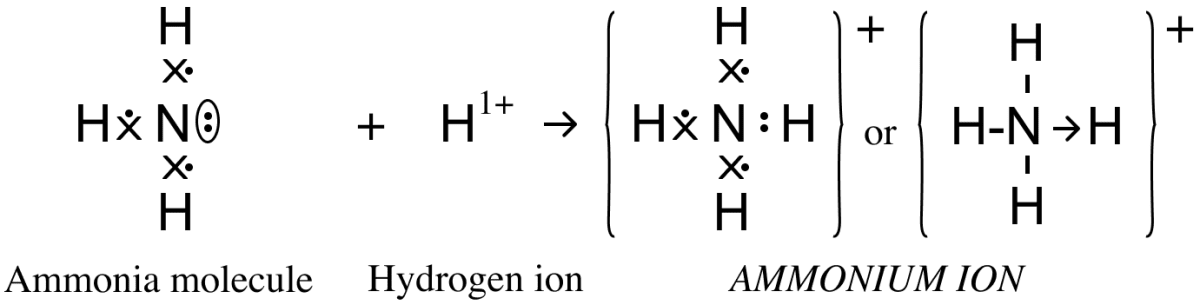(b) A molecule of nitrogen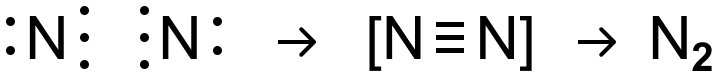#### Question 5(iii)

Give a balanced chemical equation for the following conversions with conditions:

(a) Ethene from ethanol

(b) Ethyne from calcium carbide

(c) Monochloromethane from methane

(a) Ethene from ethanol

$\underset{\text{ ethyl alcohol}}{\text{C}_2\text{H}_5\text{OH}} \xrightarrow[170\degree\text{C}]{\text{Conc. H}_2\text{SO}_4\text{[excess]}} \underset{ \text{ethene}}{\text{C}_2\text{H}_4} + \text{H}_2\text{O}\$

(b) Ethyne from calcium carbide

$\underset{\text{calcium carbide}}{\text{CaC}_2} + \underset{\text{water}}{2\text{H}_2\text{O}} \longrightarrow \underset{\text{ethyne}}{\text{C}_2\text{H}_2} + \underset{\text{calcium hydroxide}}{\text{Ca(OH)}_2}$

(c) Monochloromethane from methane

$\underset{\text{ methane} }{\text{CH}_4} + \text{Cl}_2 \xrightarrow[\Delta]{\text{diffused sunlight}} \underset{\text{monochloromethane}}{\text{CH}_3\text{Cl}} + \text{HCl}$

#### Question 5(iv)

Study the following observations and name the anions present in each of the reactions.

(a) When a crystalline solid 'P' is warmed with concentrated H2SO4 and copper turnings a reddish brown gas is released.

(b) When few drops of dilute sulphuric acid is added to Salt 'R' and heated, a colourless gas is released which turns moist lead acetate paper silvery black.

(c) When few drops of barium nitrate solution is added to the salt solution 'Q', a white precipitate is formed which is insoluble in HCl.

(a) Nitrate [NO3-]

(b) Sulphide [S2-]

(c) Sulphate [SO42-]

#### Question 6(i)

Define / State:

(a) Electronegativity

(b) Gay-Lussac's Law of combining volumes

(a) Electronegativity — The tendency of an atom in a molecule to attract the shared pair of electrons towards itself is called its electronegativity.

(b) Gay-Lussac's Law of combining volumes — When gases react, they do so in volumes which bear a simple ratio to one another, and to the volume of the gaseous product, provided that all the volumes are measured at the same temperature and pressure.

#### Question 6(ii)

The Empirical formula of an organic compound is CHCl2.

If its relative molecular mass is 168, what is its molecular formula?

[At. Wt. C = 12, H = 1, Cl = 35.5]

Empirical formula is CHCl2

Empirical formula weight = 12 + 1 + 2[35.5] = 12 + 1 + 71 = 84

Molecular weight = 168

$\text{n} = \dfrac{\text{Molecular weight}}{\text{Empirical formula weight}} \\[0.5em] = \dfrac{168}{84} = 2$

So, molecular formula = 2(CHCl2) = C2H2Cl4

#### Question 6(iii)

Choose the substances given in the box below to answer the following questions:

 Iron Magnesium sulphite Zinc Sodium sulphide Lead Ferric chloride Copper Ferrous sulphate

(a) The metal that will not produce hydrogen gas when reacted with dilute acids.

(b) The compound that will produce sulphur dioxide gas when reacted with dilute HCl.

(c) The solution of this compound produces dirty green precipitate with NaOH.

(a) Copper

(b) Sodium Sulphite

(c) Ferrous Sulphate

#### Question 6(iv)

State one relevant observation for each of the following:

(a) To the copper nitrate solution, initially few drops of sodium hydroxide solution is added and then added in excess.

(b) Burning of ammonia in excess of oxygen.

(c) Dry ammonia gas is passed over heated PbO.

(a) When a few drops of sodium hydroxide solution are added to the copper nitrate solution, light blue coloured ppt. of Cu(OH)2 is obtained which is insoluble with excess NaOH.

(b) Ammonia burns in the atmosphere of excess oxygen with a greenish yellow flame, forming nitrogen and water vapour.

(c) Buff yellow PbO is reduced to greyish metallic lead.

#### Question 7(i)

Name the following:

(a) Organic compounds with same molecular formula but different structural formula.

(b) Group of organic compounds where the successive members follow a regular structural pattern, successive compounds differ by a 'CH2' group.

(a) Isomers

(b) Homologous series

#### Question 7(ii)

Give reason for the following:

(a) Ionisation potential decreases down a group.

(b) Ionic compounds do not conduct electricity in solid state.

(a) On moving down the group, atomic size, as well as, nuclear charge increases. However, the effect of increase in atomic size dominates over the effect of increase in nuclear charge. Hence, ionization potential decreases down the group.

(b) There is a strong electrostatic force of attraction between the oppositely charged ions of the ionic compounds that keeps ions in fixed position in solid state. Due to this immobility of ions, ionic compounds do not conduct electricity in solid state.

#### Question 7(iii)

Calculate:

(a) The percentage of phosphorus in the fertilizer super phosphate Ca(H2PO4)2 correct to 1 decimal point.

[At. Wt. H=1, P=31, O=16, Ca=40]

(b) Write the empirical formula of C8H18

(a) Molecular weight of Ca(H2PO4)2
= 40 + 2[2(1) + 31 + 4(16)]
= 40 + 2[2 + 31 + 64]
= 40 + 2
= 40 + 194
= 234 g

234 g of Ca(H2PO4)2 contains 62 g of P

∴ 100 g of Ca(H2PO4)2 will contain = $\dfrac{62}{234}$ x 100 = 26.49% = 26.5%

Hence, 26.5% phosphorous is present in superphosphate Ca(H2PO4)2

(b) Given,

Molecular Formula = C8H18 = (C4H9)2

Molecular formula = n[Empirical formula]

∴ n = 2

Hence, Empirical formula = C4H9

#### Question 7(iv)

Answer the following questions with reference to electrorefining of copper:

(a) What is the anode made of?

(b) What do you observe at the cathode?

(c) Write the reaction taking place at the cathode.

(a) Impure block of copper.

(b) Pure copper is deposited on the thin sheet of pure copper placed at the cathode.

(c) Cu2+ + 2e- ⟶ Cu

#### Question 8(i)

Arrange the following according to the instructions given in brackets:

(a) C2H2, C3H6, CH4, C2H4 (In the increasing order of the molecular weight)

(b) Cu2+, Na+, Zn2+, Ag+ (The order of Preferential discharge at the cathode)

(a) Molecular weight of C2H2 = 2 + 2 = 26

Molecular weight of C3H6 = 3 + 6 = 42

Molecular weight of CH4 = 12 + 4 = 16

Molecular weight of C2H4 = 2 + 4 = 28

Hence, increasing order of molecular weights is :

CH4 < C2H2 < C2H4 < C3H6

(b) Ag+, Cu2+, Zn2+, Na+

#### Question 8(ii)

Differentiate between the following pairs based on the criteria given in the brackets:

(a) Cane sugar and hydrated copper sulphate [using concentrated H2SO4]

(b) Sulphuric acid and hydrochloric acid [type of salts formed]

(a) Conc. H2SO4 dehydrates sucrose to a black spongy charged mass of carbon sugar charcoal.

$\text{C}_{12}\text{H}_{22}\text{O}_{11}\xrightarrow{\text{Conc. H}_2\text{SO}_4} \underset{\text{sugar charcoal}}{12\text{C}} + \text{11H}_2\text{O}$

The blue coloured hydrous copper sulphate changes to white anhydrous copper sulphate as the water of crystallization is removed.

$\text{CuSO}_4\text{.5H}_2\text{O} \xrightarrow{\text{Conc. H}_2\text{SO}_4} \underset{\text{[White Anhydrous]}}{\text{CuSO}_4} + 5\text{H}_2\text{O}$

(b) Sulphuric acid forms sulphate salts whereas hydrochloric acid forms chloride salts.

#### Question 8(iii)

Convert the following reactions into a balanced chemical equation:

(a) Ammonia to nitric oxide using oxygen and platinum catalyst.

(b) Sodium hydroxide to sodium sulphate using sulphuric acid.

(c) Ferrous sulphide to hydrogen sulphide using hydrochloric acid.

(a) Ammonia to nitric oxide using oxygen and platinum catalyst.

$4\text{NH}_3 + 5\text{O}_2 \xrightarrow[800 \degree \text{C}]{\text{Pt}.} 4\text{NO} + 6\text{H}_2\text{O} + \Delta$

(b) Sodium hydroxide to sodium sulphate using sulphuric acid.

2NaOH + H2SO4 ⟶ Na2SO4 + 2H2O

(c) Ferrous sulphide to hydrogen sulphide using hydrochloric acid

FeS + 2HCl ⟶ FeCl2 + H2S

#### Question 8(iv)

Choose the answer from the list which fits in the description:

[CCl4, PbO, NaCl, CuO, NH4Cl]

(a) A compound which undergoes thermal dissociation.

(b) An amphoteric oxide.

(c) A compound which is a non-electrolyte.